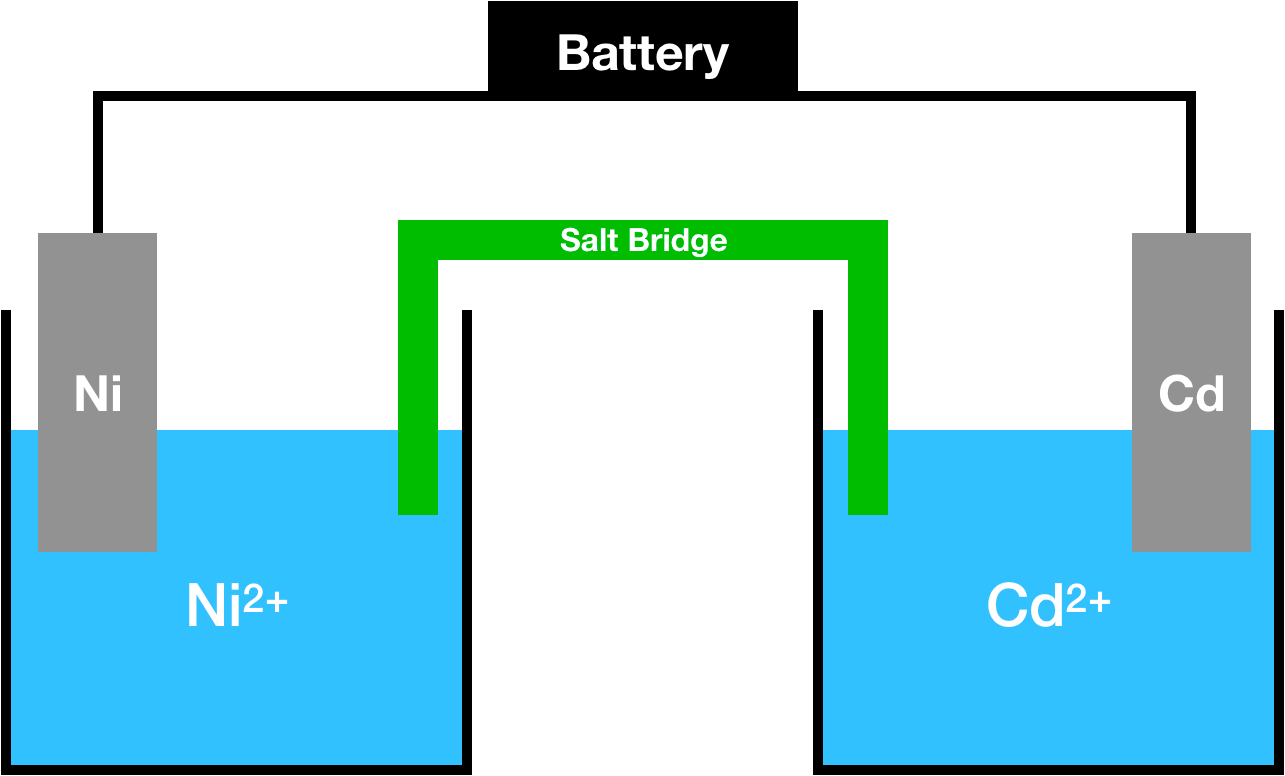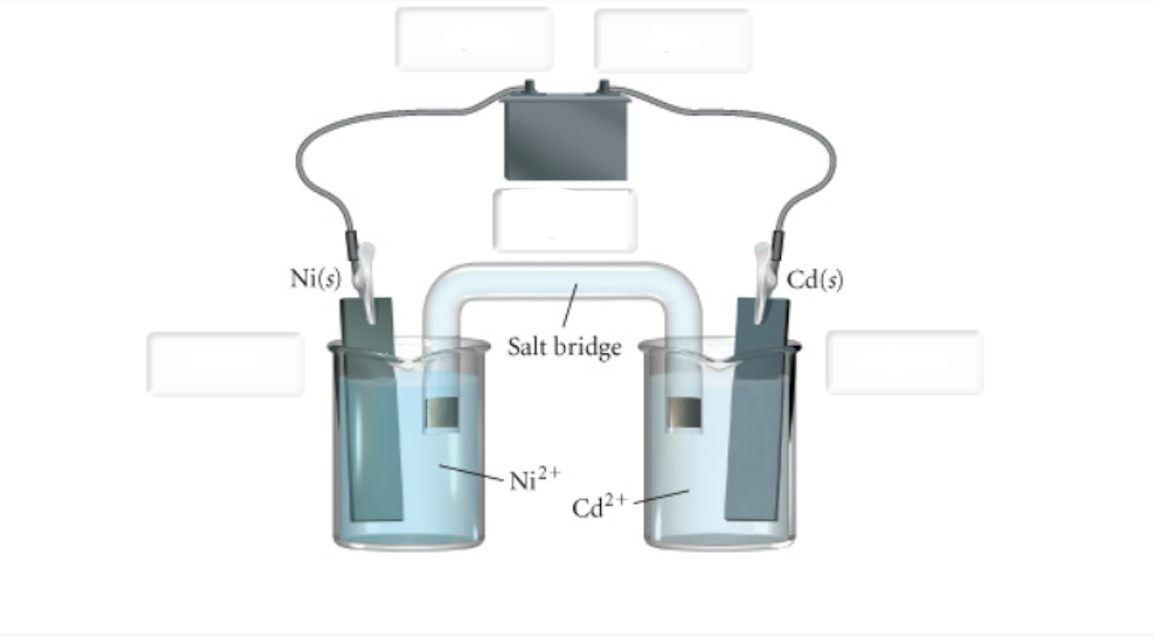# Problem: a. Label the anode and the cathode. Indicate the direction of electron flow. Label the terminals on the battery as positive or negative.b. Indicate the half-reaction occurring at Anode. Express your answer as a chemical equation. Identify all of the phases in your answer.c. Indicate the half-reaction occurring at Cathode. Express your answer as a chemical equation. Identify all of the phases in your answer.d. Calculate the minimum voltage necessary to drive the reaction. Express your answer using two significant figures.

###### FREE Expert Solution
98% (247 ratings)
###### FREE Expert Solution

We’re given the following electrochemical cell:Since a battery is given, this is an electrolytic cell, where a nonspontaneous process is occurring. Since the process is nonspontaneous, an outside energy source (battery) provides energy for it to occur.

98% (247 ratings)###### Problem Details

a. Label the anode and the cathode. Indicate the direction of electron flow. Label the terminals on the battery as positive or negative.

d. Calculate the minimum voltage necessary to drive the reaction. Express your answer using two significant figures.What scientific concept do you need to know in order to solve this problem?

Our tutors have indicated that to solve this problem you will need to apply the Galvanic Cell concept. You can view video lessons to learn Galvanic Cell. Or if you need more Galvanic Cell practice, you can also practice Galvanic Cell practice problems.

What is the difficulty of this problem?

Our tutors rated the difficulty ofa. Label the anode and the cathode. Indicate the direction o...as high difficulty.

How long does this problem take to solve?

Our expert Chemistry tutor, Dasha took 9 minutes and 24 seconds to solve this problem. You can follow their steps in the video explanation above.

What professor is this problem relevant for?

Based on our data, we think this problem is relevant for Professor Hayden's class at OAKLAND.